# Radiation Pattern Above A Half Space Medium

As mentioned earlier when discussing boundary conditions and computational domain, you can use CPML boundary conditions with zero offsets to model a structure with infinite lateral extents. At the end of the FDTD simulation, the far fields are calculated using the near-field-to-far-field transformation. This calculation requires the dyadic Green's function of the background structure. By default, the FDTD engine uses the free space dyadic Green's function for the far field calculation. In general, the FDTD Module features dyadic Green's functions for four scenarios:

1. Free space background
2. Free space background terminated in an infinite PEC ground plane at the bottom
3. Free space background terminated in an infinite PMC ground plane at the bottom
4. Free space background terminated in an infinite dielectric half-space medium

In other words, EM.Cube's FDTD Module can calculate the far field radiation pattern of a structure in the presence of any of the above four background structure types. In the case of an infinite PEC or PMC ground plane at the bottom of the domain with a zero -Z offset, it is assumed that the PEC or PMC planes extend laterally to the infinity even though your structure has a finite domain. In this case, EM.Cube automatically uses the correct dyadic Green's function and calculates the radiation pattern of a half-space structure. The far fields for observation in the lower half-space (0 ≤ θ ≤ 90°) are set equal to zero. In the fourth case, i.e. an infinite half-space ground (or a dielectric medium in general), your computational domain must end in a dielectric layer with a CPML -Z boundary. The -Z domain offset must be set equal to zero. You also have to make sure that the lateral domain offset values along the ±X and ±Y directions are set equal to zero, too. In addition, you have to specify the permittivity εr and electric conductivity σ of the terminating medium. You can set these parameters from FDTD Module's Far Field Background Medium dialog. To access this dialog, open the radiation pattern dialog and click the button labeled Background... From this dialog, you can also set the Z-coordinate of the top of the terminating half-space medium. When the bottom of your computational domain is terminated by an infinite PEC or PMC plane, the Z-coordinates of the ground plane and the bottom face of the computational domain are identical. However, when your domain is terminated in a dielectric medium, you will typically have a dielectric layer beneath your physical structure. In that case, you may want to set the Z-coordinate of the top of that dielectric layer as the position of the interface between the free space and the lower dielectric half-space.

Note that the current version of EM.Cube's FDTD Module does not calculate the far-field Green's function of a laterally infinite, conductor-backed, dielectric substrate with a finite layer thickness. For problems of this type, you should use EM.Cube's Planar Module. In FDTD, either your PEC/PMC ground can be infinite or you can assume a dielectric half-space ground. Also, note that when infinite lateral dimensions are not required, like in the case of patch antennas with a finite substrate and finite ground, FDTD is the method of choice, as the planar MoM method cannot handle such cases.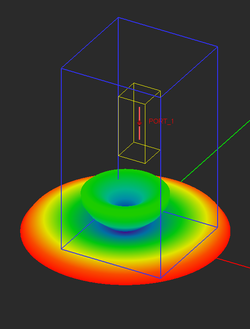Radiation pattern of a vertical short dipole above PEC ground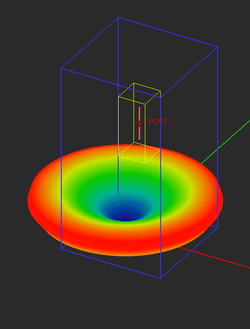Radiation pattern of a vertical short dipole above PMC ground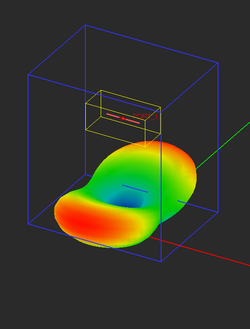A horizontal short dipole above a PEC ground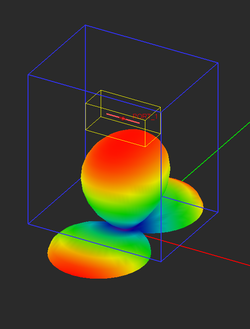a horizontal short dipole above a PMC ground. The bottom (-Z) boundary is set to PEC or PMC, even though it is not visible.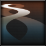More Articles Related To: EM.Tempo Advanced Simulation Options: Advanced CPML Setup - Radiation Pattern Above A Half Space Medium - Advanced Meshing in EM.Tempo - EM.Tempo Acceleration Tutorials: FDTDLesson1 - FDTDLesson2 - FDTDLesson3 - FDTDLesson4